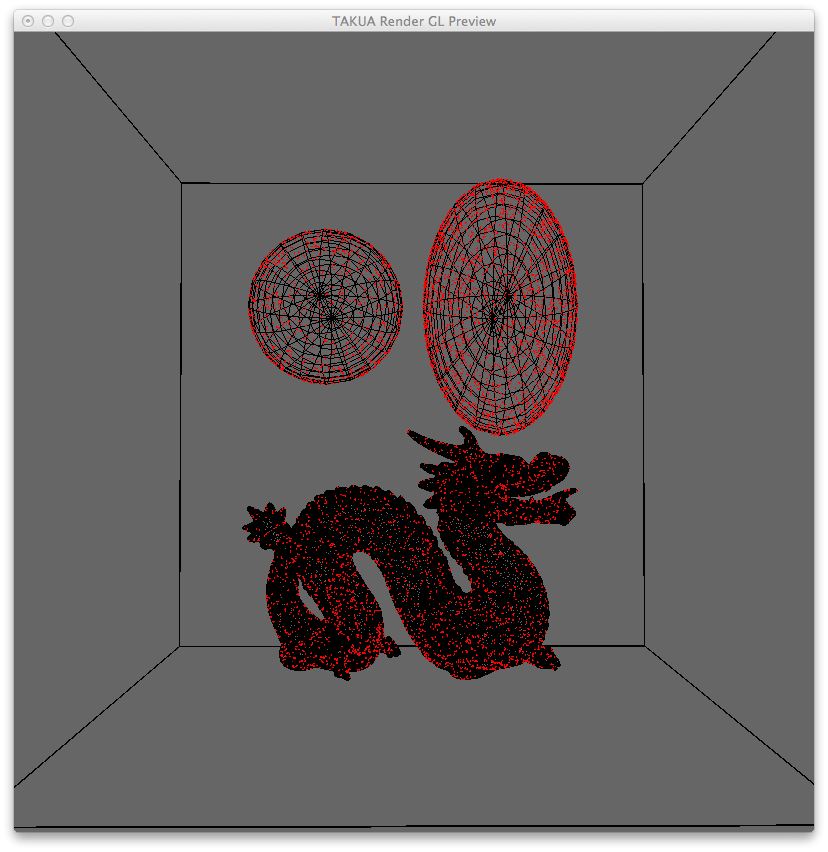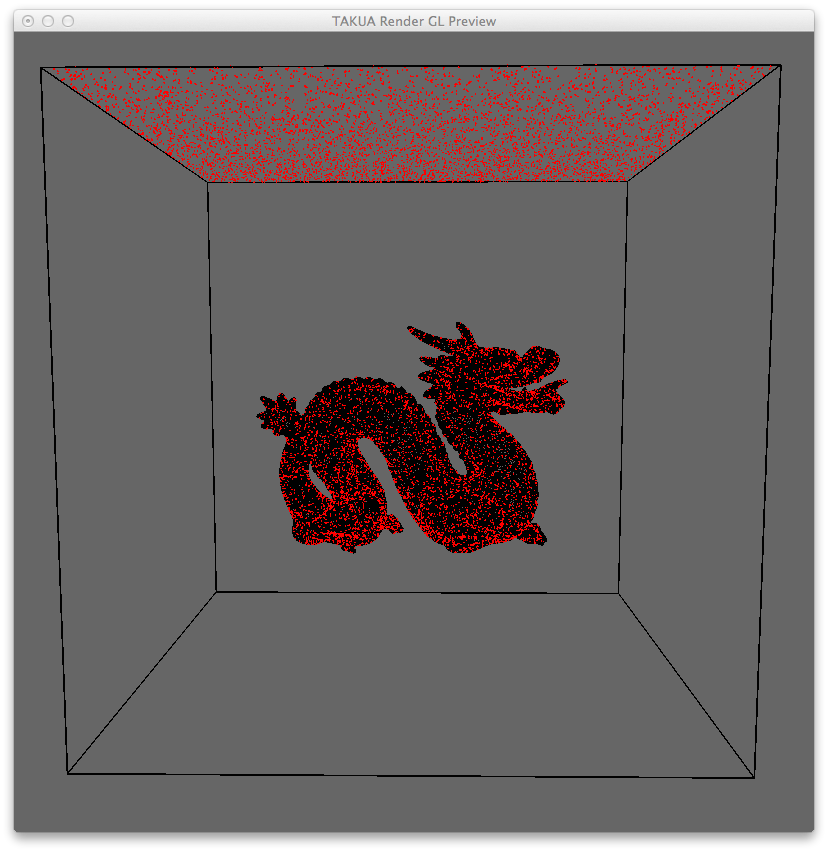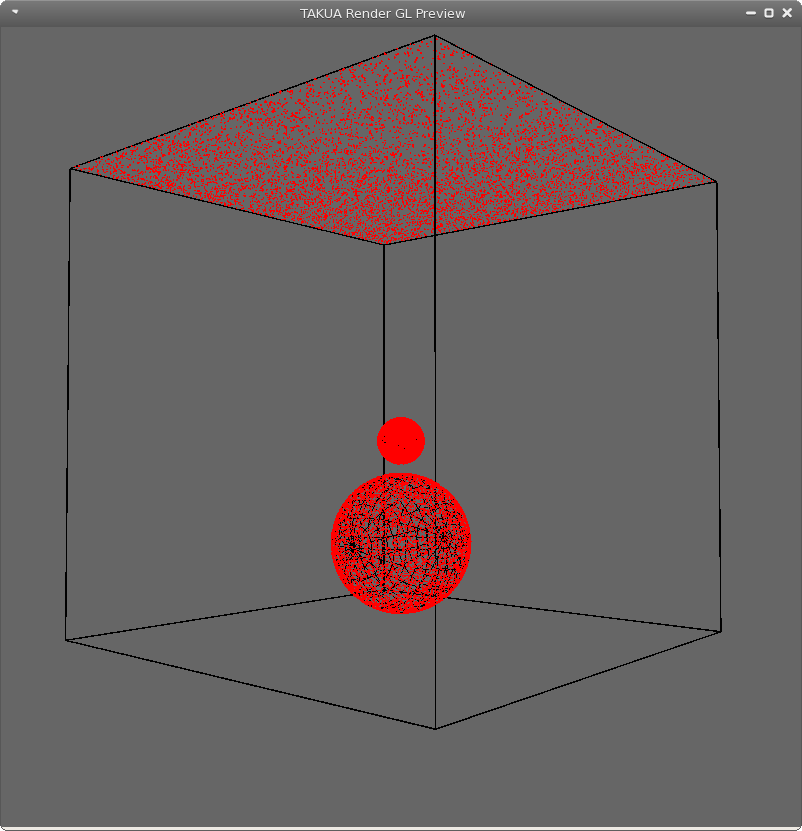## Random Point Sampling On Surfaces

Just a heads up, this post is admittedly more of a brain dump for myself than it is anything else.

A while back I implemented a couple of fast methods to generate random points on geometry surfaces, which will be useful for a number of applications, such as direct lighting calculations involving area lights.The way I’m sampling random points varies by geometry type, but all methods are pretty simple. Right now the system is implemented such that I can give the renderer a global point density to follow, and points will be generated according to that density value. This means the number of points generated on each piece of geometry is directly linked to the geometry’s surface area.

For spheres, the method I use is super simple: get the surface area of the sphere, generate random UV coordinates, and map those coordinates back to the surface of the sphere. This method is directly pulled from this Wolfram Mathworld page, which also describes why the most naive approach to point picking on a sphere is actually wrong.

My approach for ellipsoids unfortunately is a bit brute force. Since getting the actual surface area for an ellipsoid is actually fairly mathematically tricky, I just approximate it and then use plain old rejection sampling to get a point.

Boxes are the easiest of the bunch; find the surface area of each face, randomly select a face weighted by the proportion of the total surface area that face comprises, and then pick a random x and y coordinate on that face. The method I use for meshes is similar, just on potentially a larger scale: find the surface area of all of the faces in the mesh and select a face randomly weighted by the face’s proportion of the total surface area. Then instead of generating random cartesian coordinates, I generate a random barycentric coordinate, and I’m done.The method that I’m using right now is purely random, so there’s no guarantee of equal spacing between points initially. Of course, as one picks more and more points, the spacing between any given set of points will converge on something like equally spaced, but that would take a lot of random points. I’ve been looking at this Dart Throwing On Surfaces Paper for ideas, but at least for now, this solution should work well enough for what I want it for (direct lighting). But we shall see!Also, as I am sure you can guess from the window chrome on that last screenshot, I’ve successfully tested Takua Render on Linux! Specifically, on Fedora!